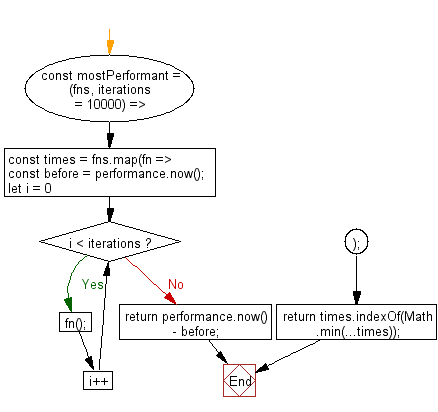# JavaScript: Get the index of the function in an array of functions which executed the fastest

## JavaScript fundamental (ES6 Syntax): Exercise-175 with Solution

Write a JavaScript program to get the index of the function in an array of functions which executed the fastest.

• Use Array.prototype.map() to generate an array where each value is the total time taken to execute the function after iterations times.
• Use the difference in performance.now() values before and after to get the total time in milliseconds to a high degree of accuracy.
• Use Math.min() to find the minimum execution time, and return the index of that shortest time which corresponds to the index of the most performant function.
• Omit the second argument, iterations, to use a default of 10000 iterations.
• The more iterations, the more reliable the result but the longer it will take.

Sample Solution:

JavaScript Code:

``````//#Source https://bit.ly/2neWfJ2
const mostPerformant = (fns, iterations = 10000) => {
const times = fns.map(fn => {
const before = performance.now();
for (let i = 0; i < iterations; i++) fn();
return performance.now() - before;
});
return times.indexOf(Math.min(...times));
};

console.log(mostPerformant([
() => {
// Loops through the entire array before returning `false`
[1, 2, 3, 4, 5, 6, 7, 8, 9, '10'].every(el => typeof el === 'number');
},
() => {
// Only needs to reach index `1` before returning false
[1, '2', 3, 4, 5, 6, 7, 8, 9, 10].every(el => typeof el === 'number');
}
])); // 1
```
```

Sample Output:

```1
```

Flowchart:Live Demo:

See the Pen javascript-basic-exercise-174-1 by w3resource (@w3resource) on CodePen.

Improve this sample solution and post your code through Disqus

What is the difficulty level of this exercise?

Test your Programming skills with w3resource's quiz.

﻿